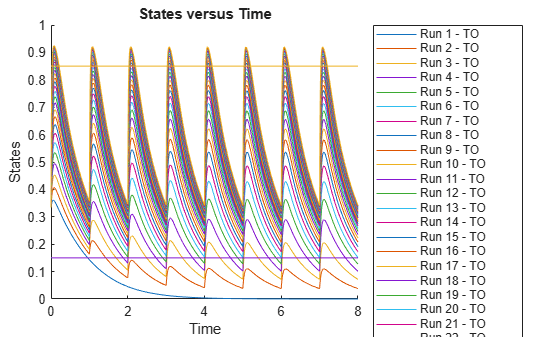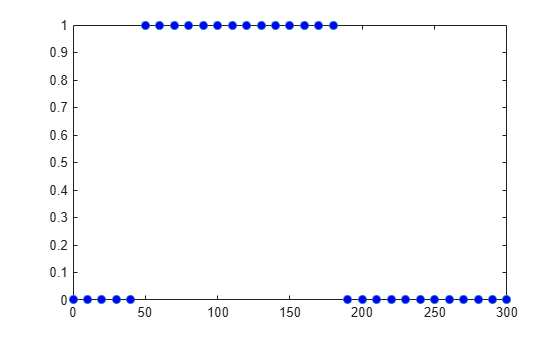# SimData

Simulation data

## Description

The `SimData` object contains simulation data, which includes time and state data, as well as metadata, such as the types and names for the logged states or the configuration set used during simulation.

You can access time data, state data, and metadata stored in the object through the object properties. Use dot notation to query the object properties or change properties that are not read-only. You can also use the `get` and `set` commands.

You can store data from multiple simulation runs as an array of `SimData` objects. You can use any `SimData` function on an array of `SimData` objects.

## Creation

Create a `SimData` object in one of the following ways.

## Properties

expand all

Simulation data, specified as an m-by-n matrix. m is the number of time steps in the simulation and n is the number of quantities and sensitivities logged during the simulation. View the corresponding time steps by accessing the `Time` property, and view the corresponding logged quantity information by accessing the `DataInfo` property.

Data Types: `double`

Number of species, compartments, parameters, and sensitivities, specified as a structure. The structure contains the fields `Species`, `Compartment`, `Parameter`, and `Sensitivity`. The default value for each field is `0`.

Data Types: `struct`

Metadata labels for simulation data, specified as an n-by-1 cell array of structures. n is the number of quantities and sensitivities logged during simulation. The ith cell contains metadata labeling the ith column of the matrix in the `Data` property.

The possible types of structures follow.

TypeFields
Species

• Type — `'species'`

• Name — Species name

• Compartment — Compartment that the species is in

• Units — Species unit

Parameter

• Type — `'parameter'`

• Name — Parameter name

• Reaction — Name of the reaction that the parameter is scoped to, or `''` if the parameter is scoped to the model

• Units — Parameter unit

Compartment

• Type — `'compartment'`

• Owner — Compartment `Owner`

• Name — Compartment name

• Units — Compartment unit

Sensitivity

• Type — `'sensitivity'`

• Name — Sensitivity name, for example, `'d[tumor_weight]/d[k2]'`

• OutputType — Sensitivity output type (`'species'` or `'parameter'`).

• OutputName — Sensitivity output name

• OutputQualifier — Sensitivity output qualifier. If the output is a species, its output qualifier is the name of the compartment that the species is in. If the output is a model-scoped parameter, its output qualifier is `''`. If the output is a reaction-scoped parameter, its output qualifier is the name of the reaction that the parameter is scoped to.

• InputType — Sensitivity input type (`'species'`, `'parameter'`, or `'compartment'`)

• InputName — Sensitivity input name

• InputQualifier — Sensitivity input qualifier. If the input is a species, its input qualifier is the name of the compartment that the species is in. If the input is a model-scoped parameter, its input qualifier is `''`. If the input is a reaction-scoped parameter, its input qualifier is the name of the reaction that the parameter is scoped to. If the input is a compartment, its input qualifier is either `''` or name of the parent compartment.

• Units — Sensitivity unit

Observable

• Scalar — Flag to indicate if the observable is scalar-valued or vector-valued.

• Expression — Observable expression

• Type — `'observable'`

• Name — Observable name

• Units — Observable unit

Data Types: `cell`

Labels for simulation data, specified as an n-by-1 cell array of character vectors. n is the number of logged quantities and sensitivities. In other words, the `DataNames` property contains the names that label the columns of the data matrix in the `Data` property.

Data Types: `cell`

Results from scalar-valued observable expressions, specified as a table. Each table variable corresponds to each observable. The name of a variable is the same as that of a scalar-valued observable. However, if the observable name is too long, it is truncated and used as the table variable name. A suffix `'_N'` is also added, where N is a positive integer. The `VariableDescriptions` property of the table contains the untruncated names.

If you specified any units for the observable, the units are copied to the `VariableUnits` property of the table.

Data Types: `table`

Results from vector-valued observable expressions, specified as a table. Each table variable corresponds to each observable. The name of a variable is the same as that of a vector-valued observable. However, if the observable name is too long, it is truncated and used as the table variable name. A suffix `'_N'` is also added, where N is a positive integer. The `VariableDescriptions` property of the table contains the untruncated names.

If you specified any units for the observable, the units are copied to the `VariableUnits` property of the table.

Data Types: `table`

Name of the simulated model, specified as a character vector.

Data Types: `char`

`SimData` object name, specified as a character vector or string.

Data Types: `char` | `string`

Additional information that you can add for the `SimData` object, specified as a character vector or string.

Data Types: `char` | `string`

Information about the simulation run that generated the simulation data, specified as a structure. The structure contains the following fields.

• `Configset` — A `struct` form of the configuration set used during simulation. The configuration set corresponds to the model active configset. The default is `[]`.

• `SimulationDate` — The date and time of simulation. The default is `''`.

• `SimulationType` — Either ```'single run'``` or `'ensemble run'`, depending on whether you create the object using `sbiosimulate` or `sbioensemblerun`. The default is `''`.

• `Variant` — A `struct` form of the variant(s) used during simulation. The default is `[]`.

Data Types: `struct`

Simulation time steps, specified as a column vector.

Data Types: `double`

Simulation time units, specified as a character vector.

If you simulate a model created using `sbiomodel`, the default `TimeUnits` value of the corresponding `SimData` object is `'second'`.

If you simulate model created using `PKModelDesign`, the default `TimeUnits` value is `'hour'`.

Data Types: `char`

Data to associate with the object, specified as any supported data type.

## Object Functions

 `addobservable` Add observable expressions to SimData `display` Display summary of SimBiology object `getdata` Get simulation data from `SimData` object `getsensmatrix` Get 3-D sensitivity matrix from `SimData` object `remove` Remove simulation data from `SimData` object using expressions `removebyname` Remove simulation data by name from `SimData` object `renameobservable` Rename observables in SimData `resample` Resample simulation data onto new time vector `select` Select simulation data from `SimData` object using expressions `selectbyname` Select simulation data by name from `SimData` object `updateobservable` Update observable expressions or units in SimData

## Examples

collapse all

`sbioloadproject gprotein.sbproj;`

Check the initial amounts of species.

`m1.Species`
```ans = SimBiology Species Array Index: Compartment: Name: Value: Units: 1 unnamed G 7000 2 unnamed Gd 3000 3 unnamed Ga 0 4 unnamed RL 0 5 unnamed L 6.022e+17 6 unnamed R 10000 7 unnamed Gbg 3000 ```

Select only the species as the states to log for simulation.

```cs = getconfigset(m1); allspecies = sbioselect(m1,'Type','species'); cs.RuntimeOptions.StatesToLog = allspecies;```

Simulate the model.

`sd = sbiosimulate(m1);`

Use the Data property of the `SimData` object `sd` to get the states at the final time point. The Data property is an m-by-n matrix, where m is the number of time steps and n is the number of quantities logged.

`finalData = sd.Data(end,:);`

Use the `DataInfo` property to get the names of logged states.

`info = sd.DataInfo;`

Loop through the species and set their initial values.

```numSpecies = length(info); vObj = addvariant(m1,'initCond'); for i = 1:numSpecies addcontent(vObj,{'species',info{i}.Name,'Value',finalData(i)}); end commit(vObj,m1);```

Verify the species initial amounts.

`m1.Species`
```ans = SimBiology Species Array Index: Compartment: Name: Value: Units: 1 unnamed G 8562.5 2 unnamed Gd 0.109565 3 unnamed Ga 1437.39 4 unnamed RL 1820.54 5 unnamed L 6.022e+17 6 unnamed R 11.1125 7 unnamed Gbg 1437.5 ```
`sbioloadproject tmdd_with_TO.sbproj`

Set the target occupancy (`TO`) as a response.

```cs = getconfigset(m1); cs.RuntimeOptions.StatesToLog = 'TO';```

Get the dosing information.

`d = getdose(m1,'Daily Dose');`

Scan over different dose amounts using a `SimBiology.Scenarios` object. To do so, first parameterize the `Amount` property of the dose. Then vary the corresponding parameter value using the `Scenarios` object.

```amountParam = addparameter(m1,'AmountParam','Units',d.AmountUnits); d.Amount = 'AmountParam'; d.Active = 1; doseSamples = SimBiology.Scenarios('AmountParam',linspace(0,300,31));```

Create a `SimFunction` to simulate the model. Set `TO `as the simulation output.

```% Suppress informational warnings that are issued during simulation. warning('off','SimBiology:SimFunction:DOSES_NOT_EMPTY'); f = createSimFunction(m1,doseSamples,'TO',d)```
```f = SimFunction Parameters: Name Value Type Units _______________ _____ _____________ ____________ {'AmountParam'} 1 {'parameter'} {'nanomole'} Observables: Name Type Units ______ _____________ _________________ {'TO'} {'parameter'} {'dimensionless'} Dosed: TargetName TargetDimension Amount AmountValue AmountUnits _______________ ___________________________________ _______________ ___________ ____________ {'Plasma.Drug'} {'Amount (e.g., mole or molecule)'} {'AmountParam'} 1 {'nanomole'} TimeUnits: day ```
`warning('on','SimBiology:SimFunction:DOSES_NOT_EMPTY');`

Simulate the model using the dose amounts generated by the `Scenarios` object. In this case, the object generates 31 different doses; hence the model is simulated 31 times and generates a `SimData` array.

```doseTable = getTable(d); sd = f(doseSamples,cs.StopTime,doseTable)```
``` SimBiology Simulation Data Array: 31-by-1 ModelName: TMDD Logged Data: Species: 0 Compartment: 0 Parameter: 1 Sensitivity: 0 Observable: 0 ```

Plot the simulation results. Also add two reference lines that represent the safety and efficacy thresholds for `TO`. In this example, suppose that any `TO` value above 0.85 is unsafe, and any `TO` value below 0.15 has no efficacy.

```h = sbioplot(sd); time = sd(1).Time; h.NextPlot = 'add'; safetyThreshold = plot(h,[min(time), max(time)],[0.85, 0.85],'DisplayName','Safety Threshold'); efficacyThreshold = plot(h,[min(time), max(time)],[0.15, 0.15],'DisplayName','Efficacy Threshold');```Postprocess the simulation results. Find out which dose amounts are effective, corresponding to the `TO` responses within the safety and efficacy thresholds. To do so, add an observable expression to the simulation data.

```% Suppress informational warnings that are issued during simulation. warning('off','SimBiology:sbservices:SB_DIMANALYSISNOTDONE_MATLABFCN_UCON'); newSD = addobservable(sd,'stat1','max(TO) < 0.85 & min(TO) > 0.15','Units','dimensionless')```
``` SimBiology Simulation Data Array: 31-by-1 ModelName: TMDD Logged Data: Species: 0 Compartment: 0 Parameter: 1 Sensitivity: 0 Observable: 1 ```

The addobservable function evaluates the new observable expression for each `SimData` in `sd` and returns the evaluated results as a new `SimData` array, `newSD`, which now has the added observable (`stat1`).

SimBiology stores the observable results in two different properties of a `SimData` object. If the results are scalar-valued, they are stored in `SimData.ScalarObservables`. Otherwise, they are stored in `SimData.VectorObservables`. In this example, the `stat1` observable expression is scalar-valued.

Extract the scalar observable values and plot them against the dose amounts.

```scalarObs = vertcat(newSD.ScalarObservables); doseAmounts = generate(doseSamples); figure plot(doseAmounts.AmountParam,scalarObs.stat1,'o','MarkerFaceColor','b')```The plot shows that dose amounts ranging from 50 to 180 nanomoles provide `TO` responses that lie within the target efficacy and safety thresholds.

You can update the observable expression with different threshold amounts. The function recalculates the expression and returns the results in a new `SimData` object array.

`newSD2 = updateobservable(newSD,'stat1','max(TO) < 0.75 & min(TO) > 0.30');`

Rename the observable expression. The function renames the observable, updates any expressions that reference the renamed observable (if applicable), and returns the results in a new `SimData` object array.

`newSD3 = renameobservable(newSD2,'stat1','EffectiveDose');`

Restore the warning settings.

`warning('on','SimBiology:sbservices:SB_DIMANALYSISNOTDONE_MATLABFCN_UCON');`

## Version History

Introduced in R2007b

expand all